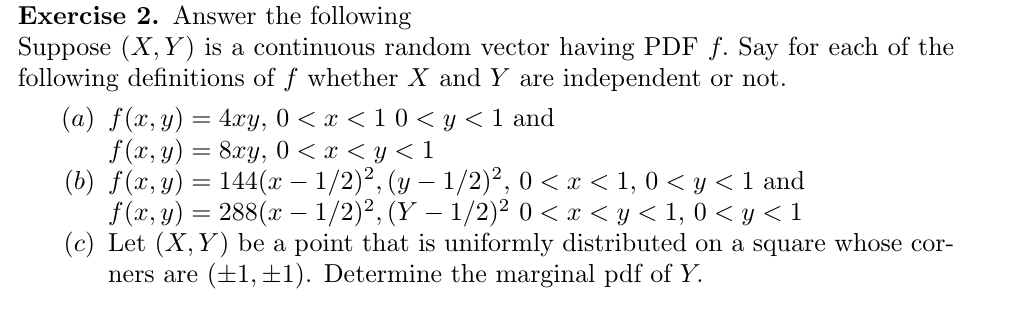# Exercise 2. Answer the followingSuppose X, Y) is a continuous random vector having PDF f. Say for each of thefollowing definitions of f whether X and Y are independent or not.(a) f(x, y) 4xy, 0 x < 10 y < 1 andf(x, y)8y 0 < x < y< 1(b) f(x, y)144(x - 1/2)2, ( 1/2)2, 0 < x < 1,0

Question

Please, help me with very detailed and self-explanatory solutions to (b) and (c) thank youhelp_outlineImage TranscriptioncloseExercise 2. Answer the following Suppose X, Y) is a continuous random vector having PDF f. Say for each of the following definitions of f whether X and Y are independent or not. (a) f(x, y) 4xy, 0 x < 10 y < 1 and f(x, y)8y 0 < x < y< 1 (b) f(x, y)144(x - 1/2)2, ( 1/2)2, 0 < x < 1,0
check_circleExpert Solution
Step 1

To work out the marginal pdf's of X and Y and to decide their independence

Step 2

(b) Working out the marginal densities of X and Y

Step 3

In this case, X and Y are indep...

### Want to see the full answer?

See Solution

#### Want to see this answer and more?

Solutions are written by subject experts who are available 24/7. Questions are typically answered within 1 hour*

See Solution
*Response times may vary by subject and question
Tagged in

### Probability